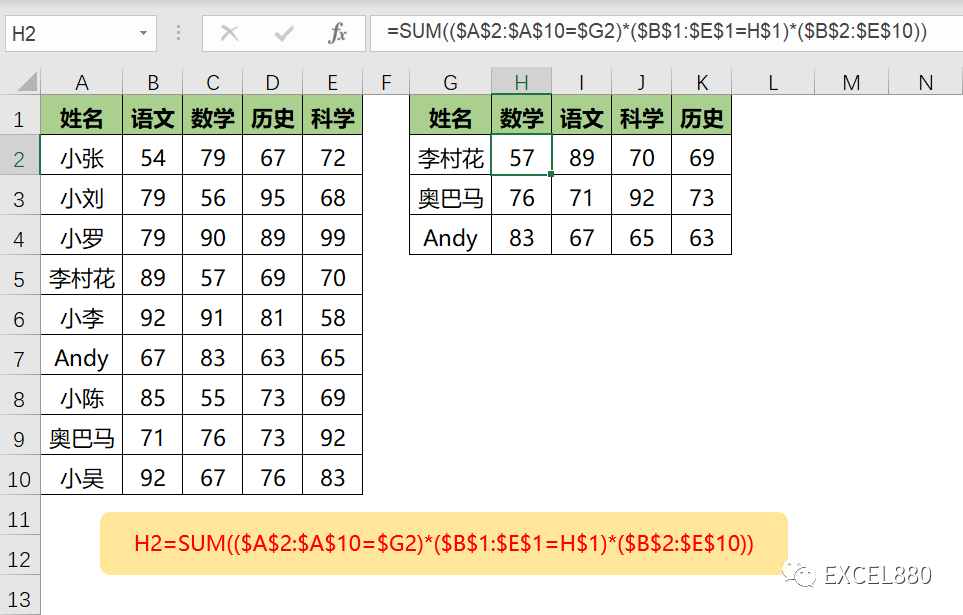# 7个Excel/WPS新函数实现的动态查询筛选函数组合，总有1个你用得着！

223 次浏览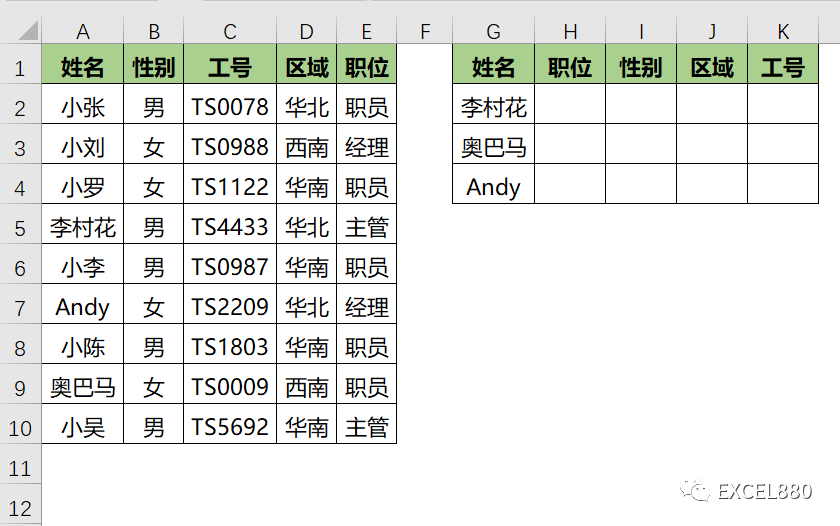VLOOKUP+MATCH

=VLOOKUP($G2,$A:$E,MATCH(H$1,$A$1:$E$1,0),0)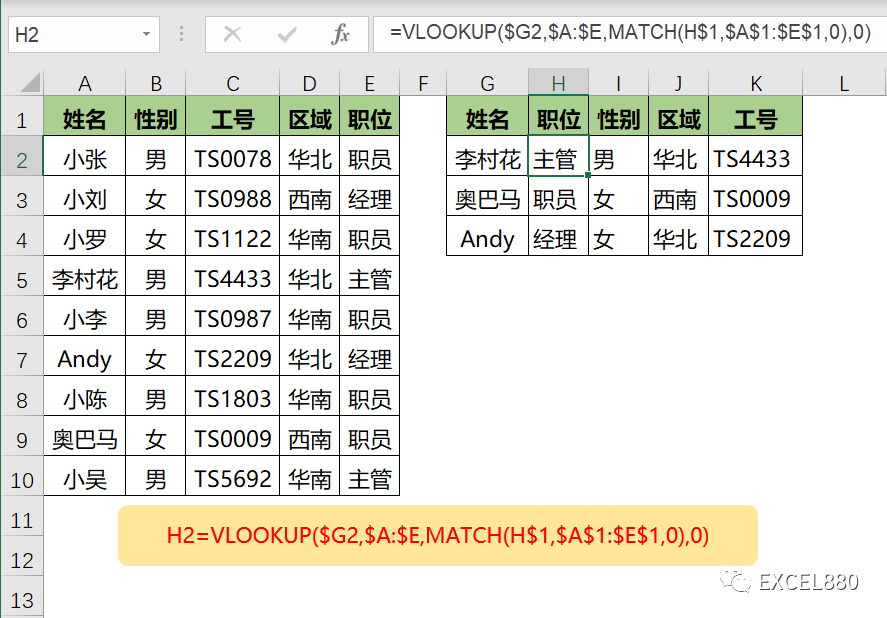INDEX+MATCH

VLOOKUP只能从左往右查，INDEX很好地弥补了这一缺陷。

=INDEX($B$2:$E$10,MATCH($G2,$A$2:$A$10,0),MATCH(H$1,$B$1:$E$1,0))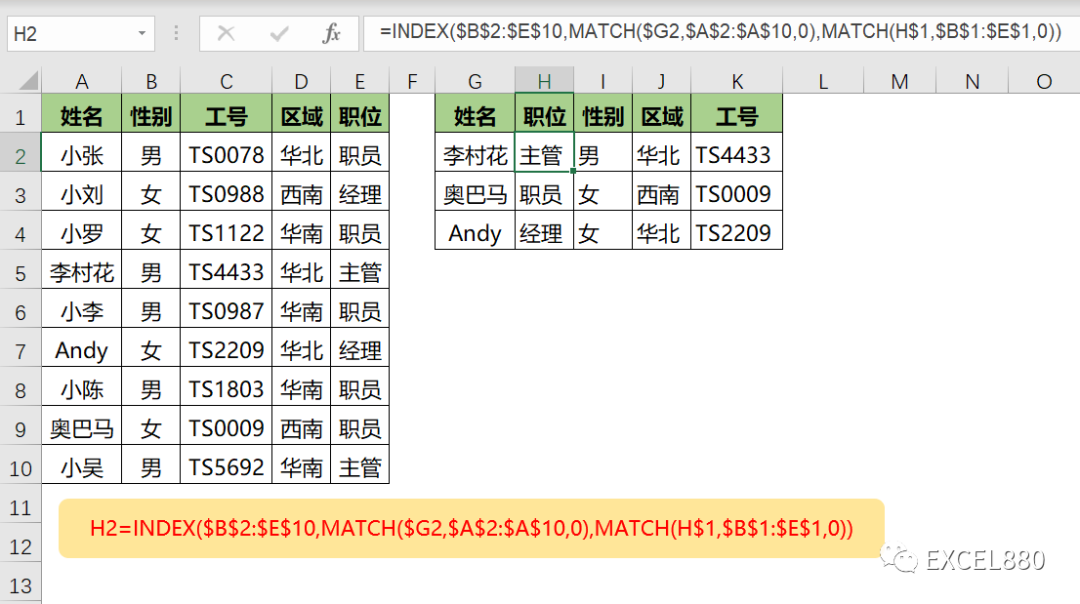XLOOKUP+XLOOKUP

=XLOOKUP($G2,$A$2:$A$10,XLOOKUP(H$1,$B$1:$E$1,$B$2:$E$10))

= XLOOKUP(H$1,$B$1:$E$1,$B$2:$E$10)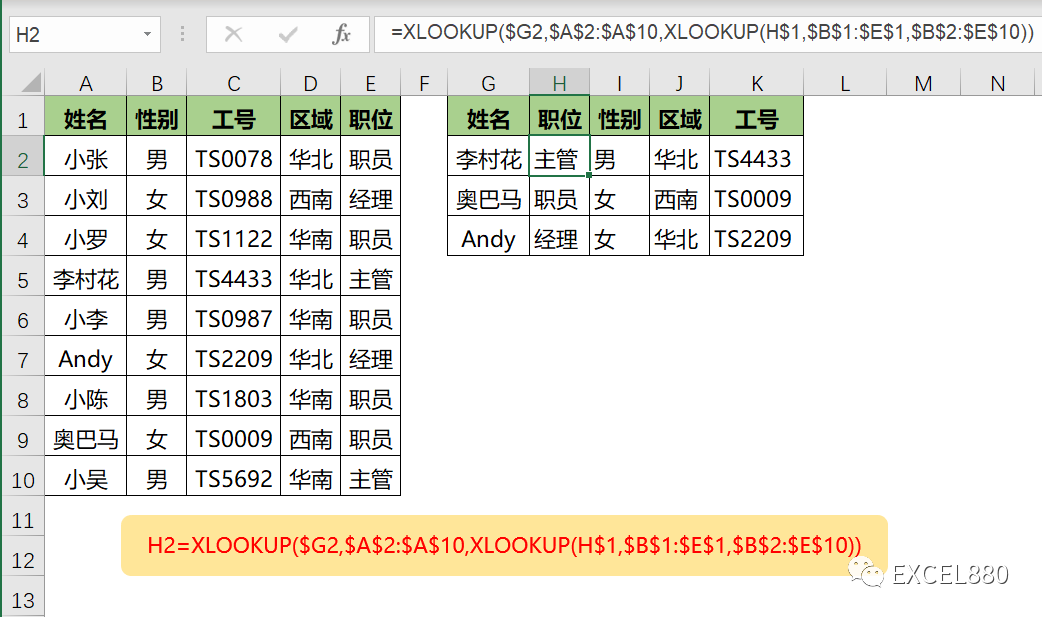XLOOKUP+FILTER XLOOKUP是查询满足条件的数据，FILTER的作用是筛选满足条件的数据。 目的都是满足条件的数据，查询出来和筛选出来，是不是有点异曲同工之妙？ =XLOOKUP($G2,$A$2:$A$10,FILTER($B$2:$E$10,$B$1:$E$1=H$1)) 把FILTER放外面，XLOOKUP放里面也是可以的，尝试一下吧。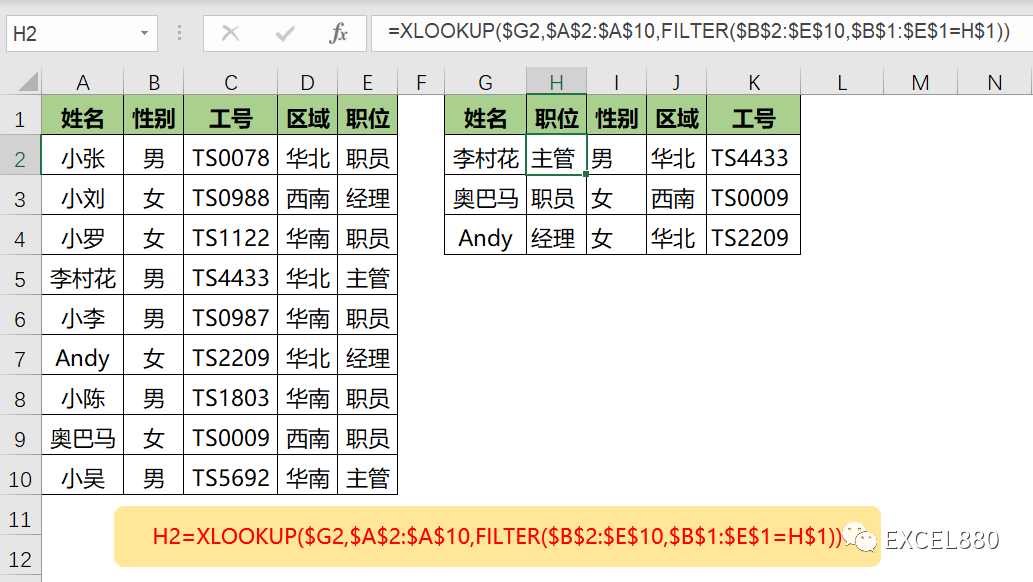FILTER+FILTER =FILTER(FILTER($B$2:$E$10,$B$1:$E$1=H$1),$A$2:$A$10=$G2) 内层FILTER先按H1的“职位”筛选，外层FILTER再按G2的“李村花”筛选。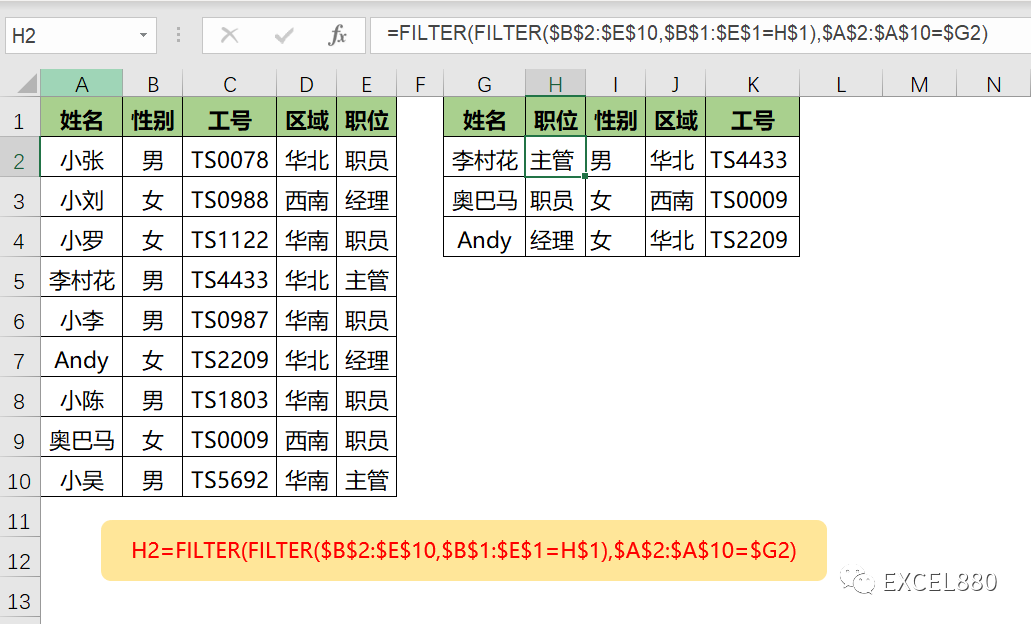TEXTJOIN+IF 用TEXTJOIN实现条件查询，卷死你的同事吧！ =TEXTJOIN(,TRUE,IF(($B$1:$E$1=H$1)*($A$2:$A$10=$G2),$B$2:$E$10,””)) IF数组的作用：如果满足两个条件则返回对应的值，否则返回空。 TEXTJOIN：将IF数组返回的数据连接起来，忽略空单元格。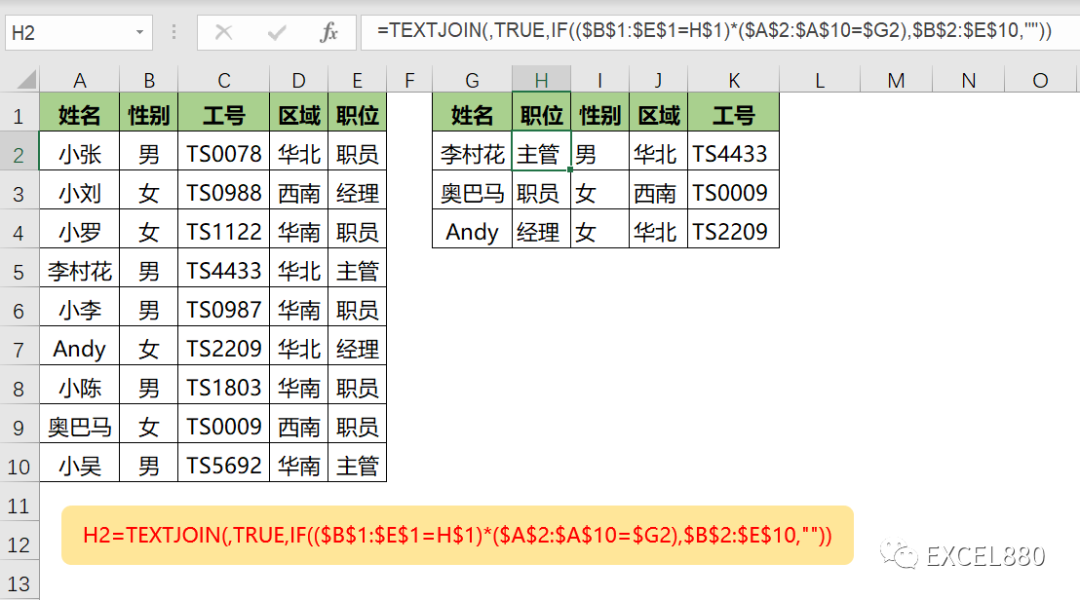SUM+数组 这种方法只适用于查询数据全部是数字的情况。 =SUM(($A$2:$A$10=$G2)*($B$1:$E$1=H$1)*($B$2:$E\$10))